# Volume calculation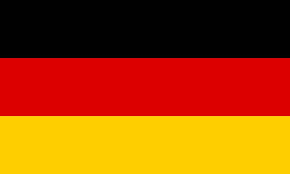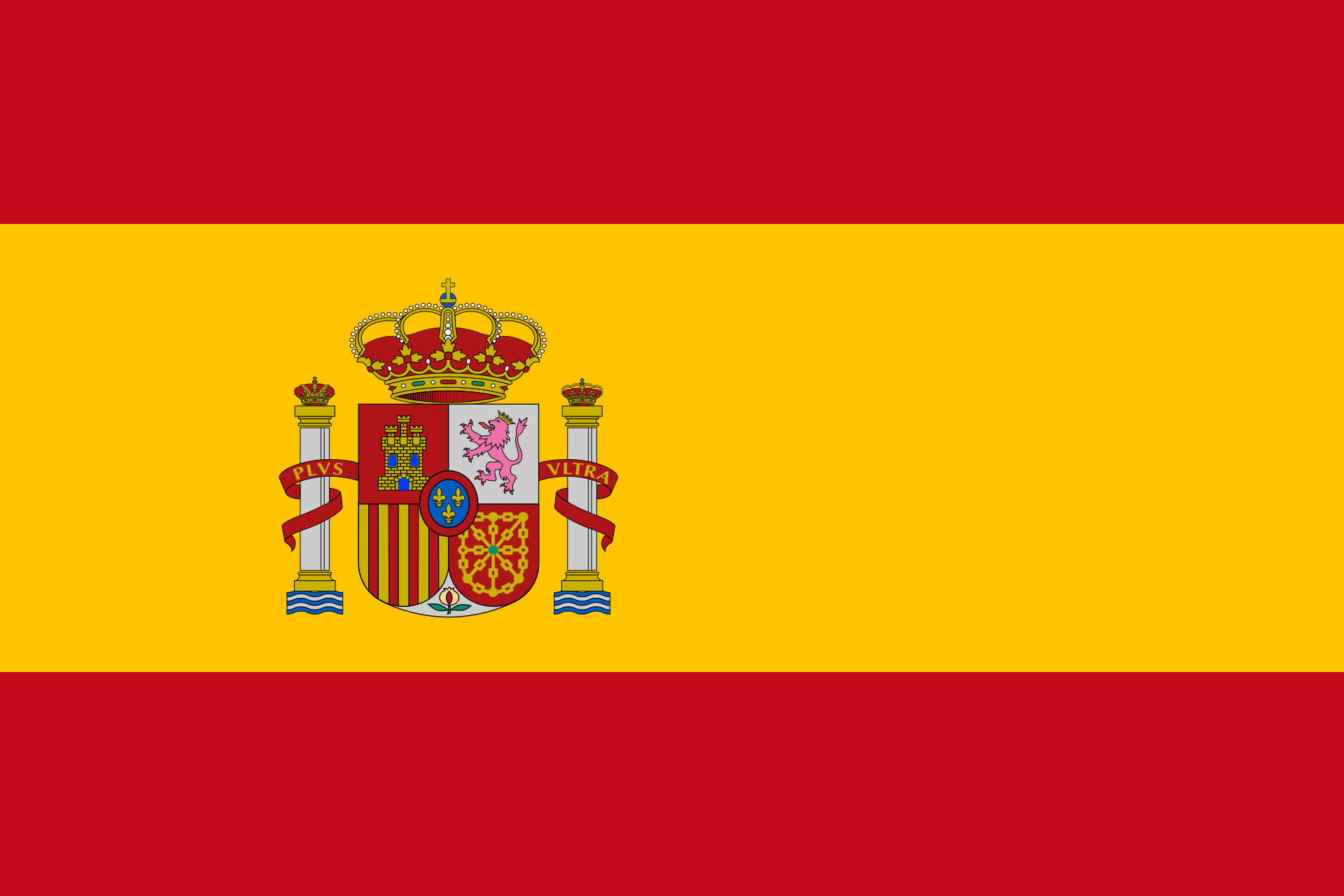Calculate a cuboid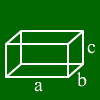Enter three values. Other values will be calculated. a: b: c: Surface: Volume: diagonal
 Calculating a cube Enter one value. Other values will be calculated. edge length Base area: Surface: Volume: Diagonal on one face Diagonal
 Calculate a prism Enter three values. Other values will be calculated.Base area: : Base area perimeter Height: Lateral area: Surface: Volume:
 Cone calculations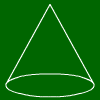radius Height: Base area: Perimeter: lateral height s: Lateral area: Surface: Volume:
 Calculating a cylinder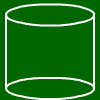Enter two values. Other values will be calculated. radius Diameter Perimeter: Base area: Height: Lateral area: Surface: Volume:

If you got a solid , the volume is the space surrounded by the of the solid.

For many solids, there are simple formulas. They are explained at the solid calculation script.